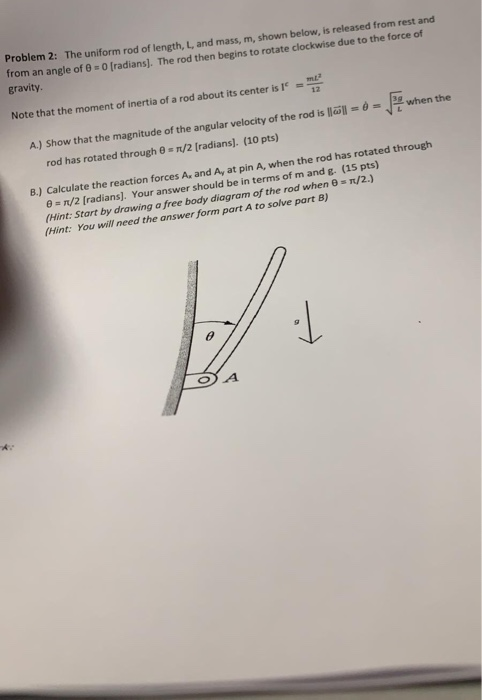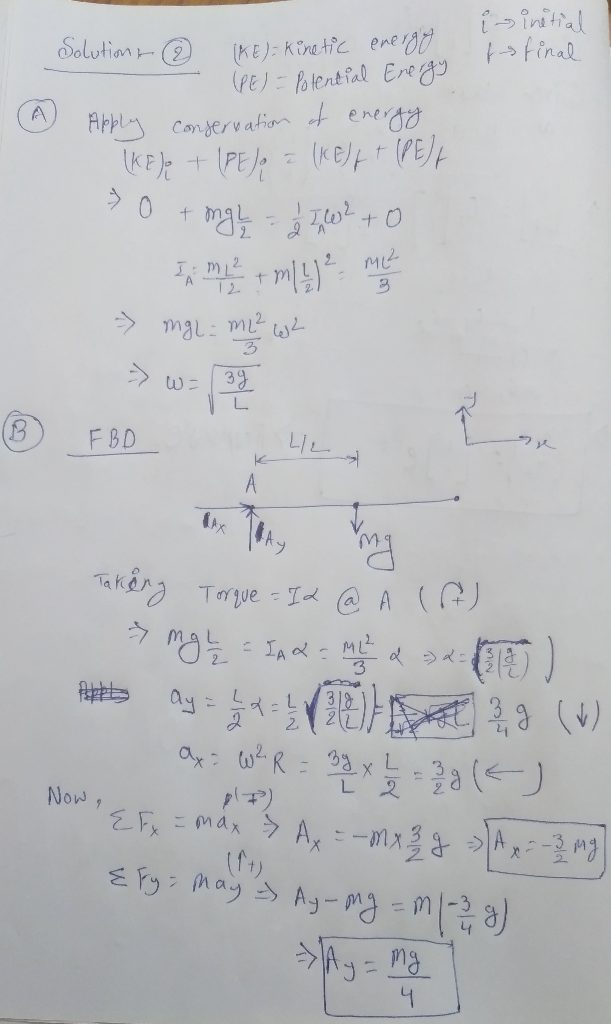# Problem 2: The uniform rod of length, L, and mass, m, shown below, is released from rest and from an angle of 8- O (radians]. The rod then begins to rotate clockwise due to the force of gravity m...Problem 2: The uniform rod of length, L, and mass, m, shown below, is released from rest and from an angle of 8- O (radians]. The rod then begins to rotate clockwise due to the force of gravity mE 12 Note that the moment of inertia of a rod about its center is when the A) Show that the magnitude of the angular velocity of the rod is löll- rod has rotated through θ s n/2 [radians]. (10 pts) forces A, and A, at pin A, when the rod has rotated through B.) Calculate the reaction e π/2 [radians (Hint: Start by drawing a free body diagram of (Hint: You will need the answer J. Your answer should be in terms of m and g. (15 pts) of the rod when rt A to solve part B) T/2.) A.##### Add Answer of: Problem 2: The uniform rod of length, L, and mass, m, shown below, is released from rest and from an angle of 8- O (radians]. The rod then begins to rotate clockwise due to the force of gravity m...
More Homework Help Questions Additional questions in this topic.